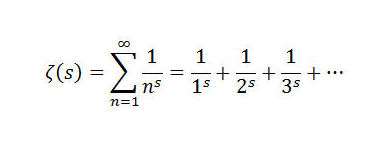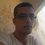# Speeding up the calculation of $\zeta(2)$.A year ago, I have posted this article, it states that if you want the correct result close by $0.01$ you should calculate about 100 terms which is huge by hand (Euler hadn't W|A to help him at the time he Calculated it ).

A way to speed up the convergence is to use $\zeta(2)=2\sum_{n>0} \frac{(-1)^{n+1}}{n^2}$, our goal is to see how much is it faster. We set : $a_m= \sum_{n=1}^{m} \frac{1}{n^2} \ \ \ \ \ , \ \ \ \ \ b_m=2\sum_{n=1}^{m} \frac{(-1)^{n+1}}{n^2}\ \ \ \ \ , m>1$

Prove or disprove that : $n \left|\frac{\zeta(2) -b_n}{\zeta(2) - a_n} \right|\to 1$ This means that $(b_n)$ converge a lot quicker than $(a_n)$.

Image credit : Recovering Lutheran blog .Note by Haroun Meghaichi
7 years ago

This discussion board is a place to discuss our Daily Challenges and the math and science related to those challenges. Explanations are more than just a solution — they should explain the steps and thinking strategies that you used to obtain the solution. Comments should further the discussion of math and science.

When posting on Brilliant:

• Use the emojis to react to an explanation, whether you're congratulating a job well done , or just really confused .
• Ask specific questions about the challenge or the steps in somebody's explanation. Well-posed questions can add a lot to the discussion, but posting "I don't understand!" doesn't help anyone.
• Try to contribute something new to the discussion, whether it is an extension, generalization or other idea related to the challenge.

MarkdownAppears as
*italics* or _italics_ italics
**bold** or __bold__ bold
- bulleted- list
• bulleted
• list
1. numbered2. list
1. numbered
2. list
Note: you must add a full line of space before and after lists for them to show up correctly
paragraph 1paragraph 2

paragraph 1

paragraph 2

[example link](https://brilliant.org)example link
> This is a quote
This is a quote
    # I indented these lines
# 4 spaces, and now they show
# up as a code block.

print "hello world"
# I indented these lines
# 4 spaces, and now they show
# up as a code block.

print "hello world"
MathAppears as
Remember to wrap math in $$ ... $$ or $ ... $ to ensure proper formatting.
2 \times 3 $2 \times 3$
2^{34} $2^{34}$
a_{i-1} $a_{i-1}$
\frac{2}{3} $\frac{2}{3}$
\sqrt{2} $\sqrt{2}$
\sum_{i=1}^3 $\sum_{i=1}^3$
\sin \theta $\sin \theta$
\boxed{123} $\boxed{123}$

Sort by:

Are there any established series that converge faster than $(bn)$?

Euler did not know all these fancy series (but the first) but he managed to get $20$ decimals using the Euler-Maclaurin summation formula which is also better than $b_n$.

- 7 years ago

Alright thanks!

- 6 years, 5 months ago

@Pranav Arora ,@Michael Lee ,@Tunk-Fey Ariawan, @Michael Mendrin (and all calculus guys). What do you think of this problem ? I can post hints if you want.

Another thing, what kind of calculus problems do prefer ? AFAIS, it is integration.

- 7 years ago